# Note: Figure is not drawn to scale

 Page 1/4 Date 28.06.2018 Size 46.47 Kb.
1   2   3   4

Area, Surface Area, & Volume
 1 Note: Figure is not drawn to scale If X = 20 units, Y = 24 units, Z = 8 units, and h = 16 units, then what is the surface area of the triangular prism shown above?

 A. 640 units2 B. 896 units2 C. 672 units2 D. 1,280 units2

 2 Note: Figure not drawn to scale. If r = 8 units and X = 5 units, then what is the volume of the cylinder shown above?

 A. 144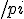cubic units B. 208cubic units C. 512cubic units D. 320cubic units

 3.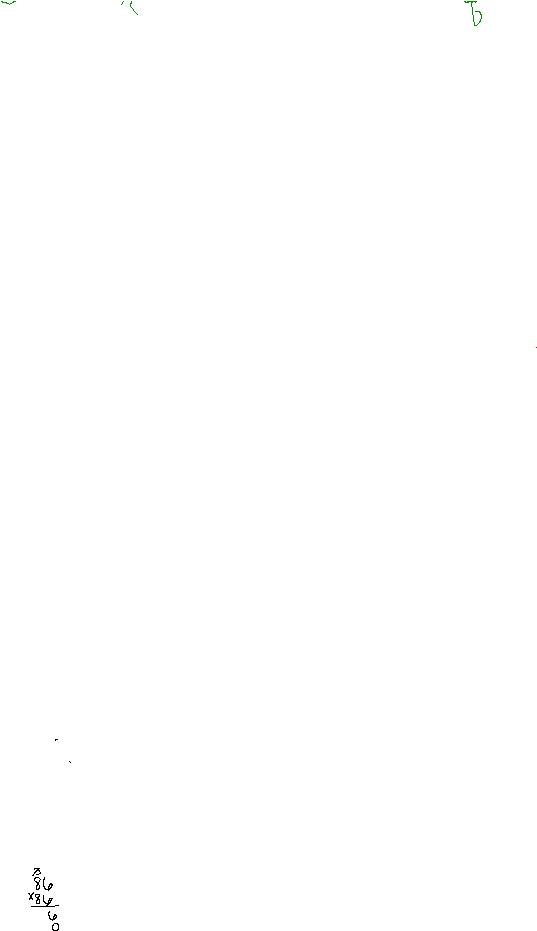Note: Figure not drawn to scale. If r = 6 units and X = 13 units, then what is the total surface area of the cylinder shown above?

 A. 936 square units B. 228 square units C. 540 square units D. 192 square units

4.

The diagram shows a walkway surrounding four identical square picnic areas.

Note: Figure not drawn to scale

If the entire diagram is a square, what is the area, in square feet (sq ft), of the walkway?

 Asquare = s2

 A. 2,500 sq ft B. 4,896 sq ft C. 7,396 sq ft D. 6,771 sq ft

 5 Note: Figure is not drawn to scale If X = 5 units, Y = 6 units, Z = 10 units, and h = 4 units, then what is the surface area of the triangular prism shown above?

 A. 208 units2 B. 114 units2 C. 104 units2 D. 184 units2

 6 Note: Figure not drawn to scale. Height has been rounded for computational ease. If h = 9 units, X = 9 units, Y = 5 units, and Z = 16 units, then what is the volume of the triangular prism shown above?

 A. 360 cubic units B. 720 cubic units C. 648 cubic units D. 413 cubic units

 7 Note: Figure not drawn to scale. If r = 4 units and X = 7 units, then what is the volume of the cylinder shown above?

 A. 64cubic units B. 72cubic units C. 88cubic units D. 112cubic units

 8 Note: Figure not drawn to scale. If r = 4 units and X = 9 units, then what is the total surface area of the cylinder shown above?

 A. 176 square units B. 88 square units C. 288 square units D. 104 square units

9.

Francisco cut a circle with a radius of 1.5 inches out of a triangle, as shown.

Note: Figure not drawn to scale

What is the area of the remaining shaded region of the triangle?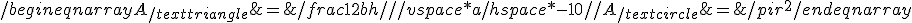A.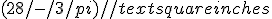B.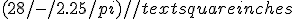C.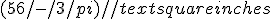D.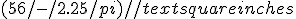10 Note: Figure is not drawn to scale If X = 6 units, Y = 17 units, and Z = 5 units, then what is the surface area of the rectangular prism shown above?

 A. 510 units2 B. 217 units2 C. 434 units2 D. 502 units2